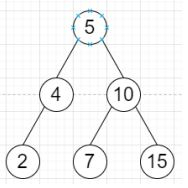# Program to create linked list to binary search tree in Python

Suppose we have a sorted linked list node of size n, we have to create a binary search tree by Taking the value of the k = floor of (n / 2) the smallest setting it as the root. Then recursively constructing the left subtree using the linked list left of the kth node. And recursively constructing the right subtree using the linked list right of the kth node.

So, if the input is like [2,4,5,7,10,15], then the output will beTo solve this, we will follow these steps−

• Define a method solve(), this will take node

• if node is null, then

• return null

• if next of node is null, then

• return a new tree node with value of node

• slow := node, fast := node

• prev := None

• while fast and next of fast are not null, do

• prev := slow

• slow := next of slow

• fast := next of next of fast

• next of prev := None

• root := a new tree node with value of slow

• left of root := solve(node)

• right of root := solve(next of slow)

• return root

Let us see the following implementation to get better understanding −

## Example

class ListNode:
def __init__(self, data, next = None):
self.val = data
self.next = next
class TreeNode:
def __init__(self, data, left = None, right = None):
self.data = data
self.left = left
self.right = right

def make_list(elements):
for element in elements[1:]:
while ptr.next:
ptr = ptr.next
ptr.next = ListNode(element)

def print_tree(root):
if root is not None:
print_tree(root.left)
print(root.data, end = ', ')
print_tree(root.right)

class Solution:
def solve(self, node):
if not node:
return None
if not node.next:
return TreeNode(node.val)
slow = fast = node
prev = None
while fast and fast.next:
prev = slow
slow = slow.next
fast = fast.next.next
prev.next = None
root = TreeNode(slow.val)
root.left = self.solve(node)
root.right = self.solve(slow.next)

return root

ob = Solution()
print_tree(root)
[2,4,5,7,10,15]
2, 4, 5, 7, 10, 15,